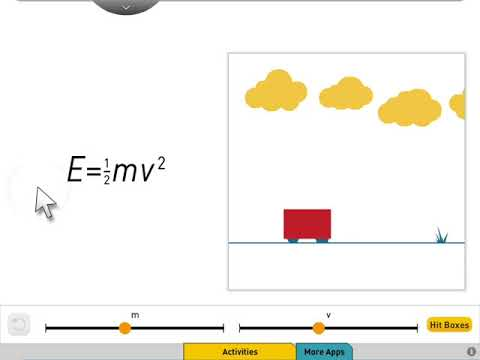# Formulas for Physics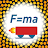5+Everyone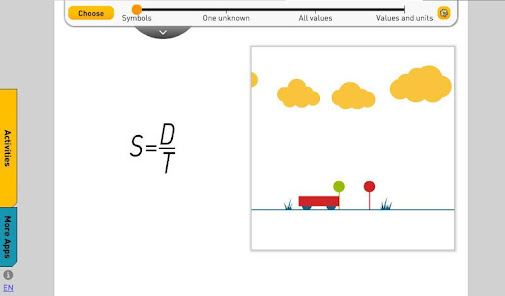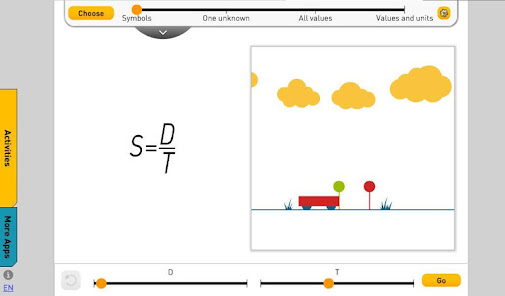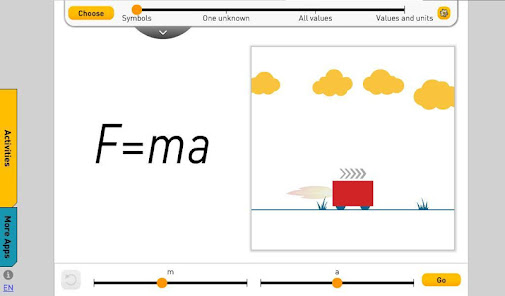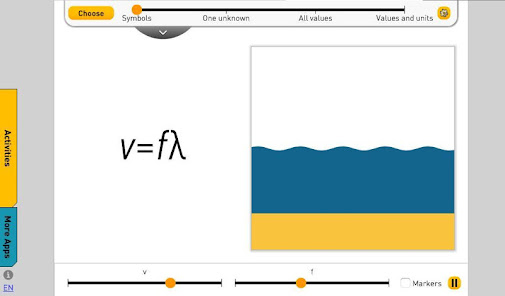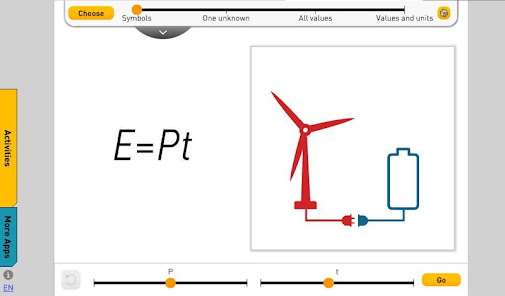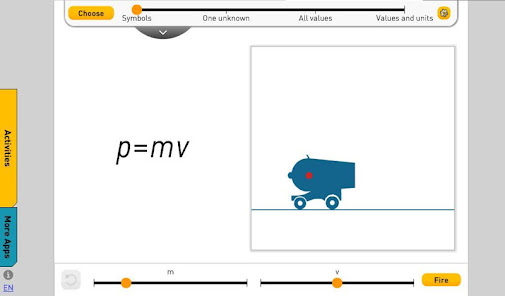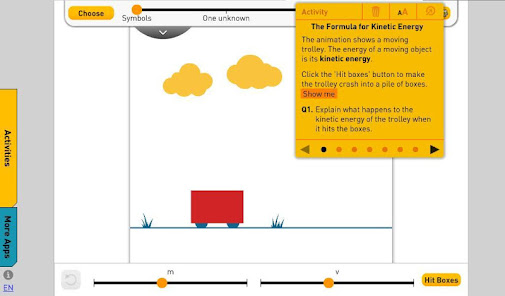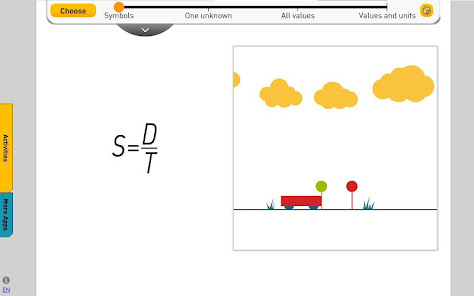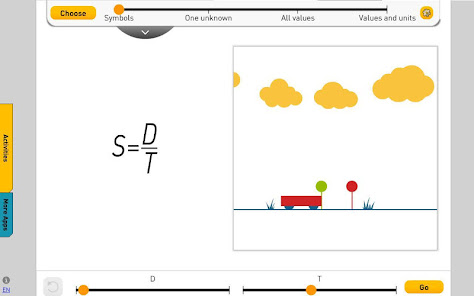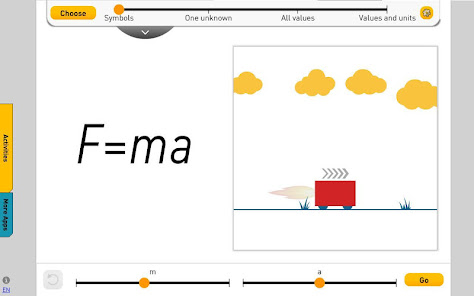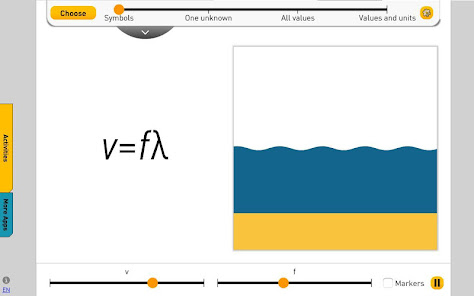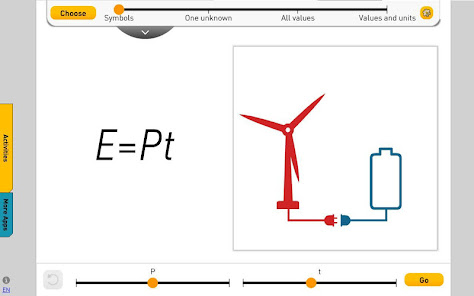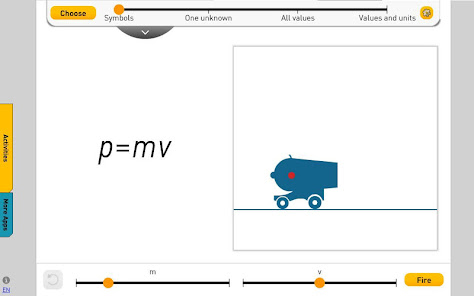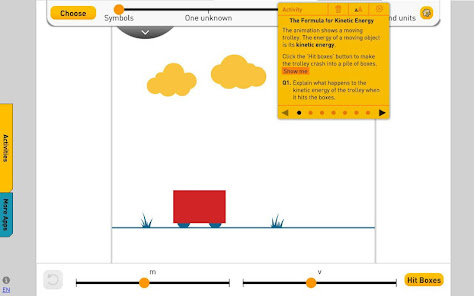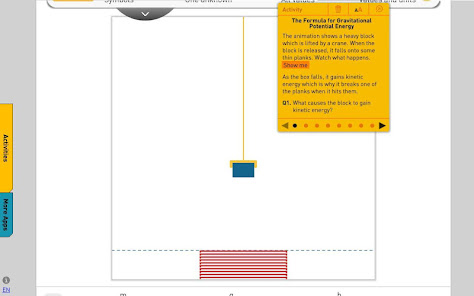Formulas for Physics gives you the perfect way to introduce physics formulas, each with a simple animation and an interactive formula. You’ll use it to cover:
• What a formula actually is.
• Substituting values.
• Units in formulas
• Simple relationships in physics, like speed, distance and time
• The ways scientists calculate energy.
• Simple circuits formulas
• Lots of other topics like pressure, momentum, acceleration
Updated on
Apr 7, 2020

## Data safety

Safety starts with understanding how developers collect and share your data. Data privacy and security practices may vary based on your use, region, and age. The developer provided this information and may update it over time.No data shared with third parties